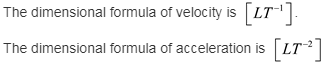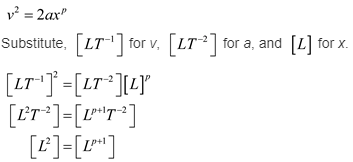# Velocity is related to acceleration and distance by the following expression: v2 = 2axp

Velocity is related to acceleration and distance by the following expression: v2 = 2axp. Find the power p that makes this equation diniensionally consistent.